# How to Develop a Random Forest Ensemble in Python

Random forest is an ensemble supervised machine learning algorithm made up of decision trees. It is used for classification and for regression as well. In Random Forest, the dataset is divided into two parts (training and testing). Based on multiple parameters, the decision is taken and the target data is predicted or classified accordingly.

Random Forest is a collection of multiple decision trees and the final result is based on the aggregated result of all the decision trees.

To better understand Random Forest, let’s take an example of the Iris Dataset. Iris dataset is by default present in the scikit-learn library of Python.

Dataset Attribute Information:

• sepal length in cm
• sepal width in cm
• petal length in cm
• petal width in cm
• class:
• Iris Setosa
• Iris Versicolour
• Iris Virginica

## Stepwise Implementation

Step 1 :

Scikit-learn Scikit-learn (Sklearn) is the most useful, robust, and free machine learning library in Python. It is an efficient tool for machine learning and statistical modeling that features various algorithms like classification, regression, clustering, random forests, k-neighbors, and dimensionality reduction.

## Python3

 `# Import scikit-learn dataset library` `from` `sklearn ``import` `datasets`   `# Load dataset` `iris ``=` `datasets.load_iris()`

Step 2:

Print the dependent and independent variables of the iris dataset and group them accordingly.

Dependent variables: The variables whose value is dependent on the other attributes of the table.

Independent variables: The variables whose value is independent of the other attributes of the table.

## Python3

 `# print the label species(setosa , ` `# versicolor , virginica )` `print``(iris.target_names)`   `# print the names of the four features` `print``(iris.features_names)`

Output: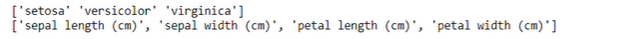Step 3:

Print the top 5 records and rename the values of setosa, versicolor, virginica as 0,1,2 for better prediction of the model.

## Python3

 `# print the iris data(top 5 records)` `print``(iris.data[``0``:``5``])`   `# print the iris labels ( 0:setosa ,` `# 1:versicolor , 2:viriginica)` `print``(iris.target)`

Output: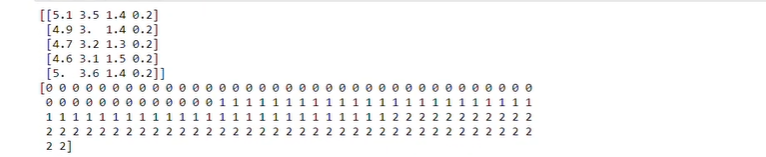Step 4:

Import pandas library of python for creating data frame of the iris dataset. Pandas are used for data cleaning and analysis. It is built on top of the Numpy Library, which is used for building various data structures and operations for manipulating numerical data and time series.

## Python3

 `# Creating a Dataframe of given iris dataset.` `import` `pandas as pd` `data ``=` `pd.DataFrame({` `    ``'sepal length'``: iris.data[:, ``0``],` `    ``'sepal width'``: iris.data[:, ``1``],` `    ``'petal length'``: iris.data[:, ``2``],` `    ``'petal width'``: iris.data[:, ``3``],` `    ``'species'``: iris.target` `})`   `data.head()`

Output: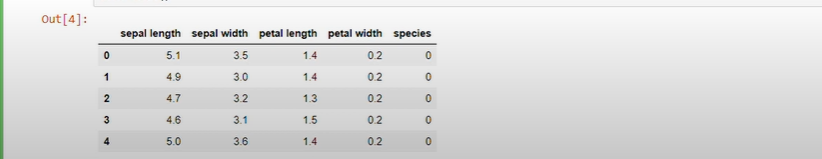Step 5:

Splitting the dataset into two parts – training and testing. The training dataset is used to train the model and the testing dataset is used to test whether the model gives accurate predictions. For performing this operation, a train_test_split package is imported from sklearn.model_selection library.

## Python3

 `# Import train_test_split function` `from` `sklearn.model_selection ``import` `train_test_split`   `X ``=` `data[[``'sepal length'``, ``'sepal width'``,` `          ``'petal length'``, ``'petal width'``]]  ``# Features` `y ``=` `data[``'species'``]  ``# Labels`   `# Split dataset into training set and test set` `X_train, X_test, y_train, y_test ``=` `train_test_split(` `    ``X, y, test_size``=``0.3``)  ``# 70% training and 30% test`

Step 6:

Now, after splitting the dataset Random Forest Algorithm is applied. For that, the RandomForestClassifier package is imported from sklearn.ensemble library and X_train(training part of Dependent variable) and y_train(training part of Independent variable) are fitted on the created model. The model is used to predict the  y_pred(independent variable) with the help of X_test(testing part of the Dependent variable).

## Python3

 `# Import Random Forest Model` `from` `sklearn.ensemble ``import` `RandomForestClassifier`   `# Create a Gaussian Classifier` `clf ``=` `RandomForestClassifier(n_estimator``=``100``)`   `# Train the model using the training sets ` `# y_pred = clf.predict(X_test)` `clf.fit(X_train, y_train)`   `y_pred ``=` `clf.predict(X_test)`

Step 7:

To check the accuracy of the model, we need to import the metrics package from the sklearn library and another way to calculate the accuracy of the model is by creating the confusion matrix.

## Python3

 `# Import scikit-learn metrics ` `# module for accuracy calculation` `from` `sklearn ``import` `metrics`   `# Number of right values predicted right ` `# and wrong values predicted wrong` `confusion_matrix ``=` `metrics.confusion_matrix(y_test, y_pred)` `print``(confusion_matrix)`   `# Model Accuracy how often is the` `# classifier correct ?` `print``(``"Accuracy : "``, metrics.accuracy_score(y_test, y_pred)`

Output: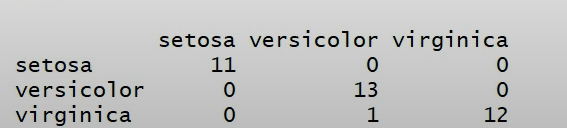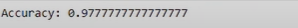Whether you're preparing for your first job interview or aiming to upskill in this ever-evolving tech landscape, GeeksforGeeks Courses are your key to success. We provide top-quality content at affordable prices, all geared towards accelerating your growth in a time-bound manner. Join the millions we've already empowered, and we're here to do the same for you. Don't miss out - check it out now!

Previous
Next